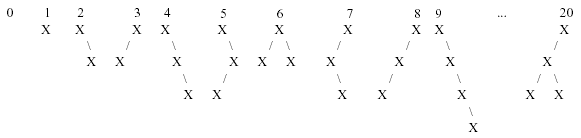Time Limit: Java: 2000 ms / Others: 2000 ms

Memory Limit: Java: 65536 KB / Others: 65536 KB

## Description

We can number binary trees using the following scheme:

The empty tree is numbered 0.
The single-node tree is numbered 1.
All binary trees having m nodes have numbers less than all those having m+1 nodes.
Any binary tree having m nodes with left and right subtrees L and R is numbered n such that all trees having m nodes numbered > n have either
Left subtrees numbered higher than L, or
A left subtree = L and a right subtree numbered higher than R.

The first 10 binary trees and tree number 20 in this sequence are shown below:Your job for this problem is to output a binary tree when given its order number.

## Input

Input consists of multiple problem instances. Each instance consists of a single integer n, where 1 <= n <= 500,000,000. A value of n = 0 terminates input. (Note that this means you will never have to output the empty tree.)

## Output

For each problem instance, you should output one line containing the tree corresponding to the order number for that instance. To print out the tree, use the following scheme:

A tree with no children should be output as X.
A tree with left and right subtrees L and R should be output as (L')X(R'), where L' and R' are the representations of L and R.
If L is empty, just output X(R').
If R is empty, just output (L')X.

## Sample Input

1
20
31117532
0

## Sample Output

X
((X)X(X))X
(X(X(((X(X))X(X))X(X))))X(((X((X)X((X)X)))X)X)

None

## Source

East Central North America 2001